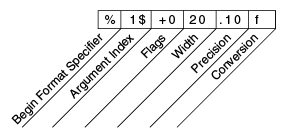Documentation

The Java™ Tutorials
Hide TOC
Trail: Essential Java Classes
Lesson: Basic I/O
Section: I/O Streams
Subsection: Scanning and Formatting

# Formatting

Stream objects that implement formatting are instances of either `PrintWriter`, a character stream class, or `PrintStream`, a byte stream class.

Note: The only `PrintStream` objects you are likely to need are `System.out` and `System.err`. (See I/O from the Command Line for more on these objects.) When you need to create a formatted output stream, instantiate `PrintWriter`, not `PrintStream`.

Like all byte and character stream objects, instances of `PrintStream` and `PrintWriter` implement a standard set of `write` methods for simple byte and character output. In addition, both `PrintStream` and `PrintWriter` implement the same set of methods for converting internal data into formatted output. Two levels of formatting are provided:

• `print` and `println` format individual values in a standard way.
• `format` formats almost any number of values based on a format string, with many options for precise formatting.

## The `print` and `println` Methods

Invoking `print` or `println` outputs a single value after converting the value using the appropriate `toString` method. We can see this in the `Root` example:

```public class Root {
public static void main(String[] args) {
int i = 2;
double r = Math.sqrt(i);

System.out.print("The square root of ");
System.out.print(i);
System.out.print(" is ");
System.out.print(r);
System.out.println(".");

i = 5;
r = Math.sqrt(i);
System.out.println("The square root of " + i + " is " + r + ".");
}
}
```

Here is the output of `Root`:

```The square root of 2 is 1.4142135623730951.
The square root of 5 is 2.23606797749979.
```

The `i` and `r` variables are formatted twice: the first time using code in an overload of `print`, the second time by conversion code automatically generated by the Java compiler, which also utilizes `toString`. You can format any value this way, but you don't have much control over the results.

## The `format` Method

The `format` method formats multiple arguments based on a format string. The format string consists of static text embedded with format specifiers; except for the format specifiers, the format string is output unchanged.

Format strings support many features. In this tutorial, we'll just cover some basics. For a complete description, see `format string syntax` in the API specification.

The `Root2` example formats two values with a single `format` invocation:

```public class Root2 {
public static void main(String[] args) {
int i = 2;
double r = Math.sqrt(i);

System.out.format("The square root of %d is %f.%n", i, r);
}
}
```

Here is the output:

```The square root of 2 is 1.414214.
```

Like the three used in this example, all format specifiers begin with a `%` and end with a 1- or 2-character conversion that specifies the kind of formatted output being generated. The three conversions used here are:

• `d` formats an integer value as a decimal value.
• `f` formats a floating point value as a decimal value.
• `n` outputs a platform-specific line terminator.

Here are some other conversions:

• `x` formats an integer as a hexadecimal value.
• `s` formats any value as a string.
• `tB` formats an integer as a locale-specific month name.

There are many other conversions.

Note:

Except for `%%` and `%n`, all format specifiers must match an argument. If they don't, an exception is thrown.

In the Java programming language, the `\n` escape always generates the linefeed character (`\u000A`). Don't use `\n` unless you specifically want a linefeed character. To get the correct line separator for the local platform, use `%n`.

In addition to the conversion, a format specifier can contain several additional elements that further customize the formatted output. Here's an example, `Format`, that uses every possible kind of element.

```public class Format {
public static void main(String[] args) {
System.out.format("%f, %1\$+020.10f %n", Math.PI);
}
}
```

Here's the output:

```3.141593, +00000003.1415926536
```

The additional elements are all optional. The following figure shows how the longer specifier breaks down into elements.Elements of a Format Specifier.

The elements must appear in the order shown. Working from the right, the optional elements are:

• Precision. For floating point values, this is the mathematical precision of the formatted value. For `s` and other general conversions, this is the maximum width of the formatted value; the value is right-truncated if necessary.
• Width. The minimum width of the formatted value; the value is padded if necessary. By default the value is left-padded with blanks.
• Flags specify additional formatting options. In the `Format` example, the `+` flag specifies that the number should always be formatted with a sign, and the `0` flag specifies that `0` is the padding character. Other flags include `-` (pad on the right) and `,` (format number with locale-specific thousands separators). Note that some flags cannot be used with certain other flags or with certain conversions.
• The Argument Index allows you to explicitly match a designated argument. You can also specify `<` to match the same argument as the previous specifier. Thus the example could have said: `System.out.format("%f, %<+020.10f %n", Math.PI);`

Previous page: Scanning
Next page: I/O from the Command Line